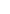LCOV - code coverage reportCurrent view: top level - py - modmath.c (source / functions) Hit Total Coverage Test: unix_coverage_v1.20.0-498-g5dbd6fc70.info Lines: 124 124 100.0 % Date: 2023-09-28 15:23:00 Functions: 46 46 100.0 % Branches: 56 56 100.0 %Function NameHit countcopysign_func 168 fabs_func 64 math_error 420 math_generic_1 1212 math_generic_2 660 mp_math_acos 68 mp_math_acosh 36 mp_math_asin 68 mp_math_asinh 44 mp_math_atan 64 mp_math_atan2 164 mp_math_atanh 40 mp_math_ceil 100 mp_math_copysign 168 mp_math_cos 68 mp_math_cosh 44 mp_math_degrees 64 mp_math_erf 44 mp_math_erfc 44 mp_math_exp 84 mp_math_expm1 32 mp_math_fabs 64 mp_math_factorial 124 mp_math_factorial_inner 1300 mp_math_floor 100 mp_math_fmod 164 mp_math_frexp 32 mp_math_gamma 52 mp_math_isclose 108 mp_math_isfinite 40 mp_math_isinf 40 mp_math_isnan 48 mp_math_ldexp 52 mp_math_lgamma 60 mp_math_log 242 mp_math_log10 52 mp_math_log2 52 mp_math_modf 32 mp_math_pow 164 mp_math_radians 64 mp_math_sin 64 mp_math_sinh 44 mp_math_sqrt 64 mp_math_tan 64 mp_math_tanh 60 mp_math_trunc 100Generated by: LCOV version 1.15-5-g462f71d